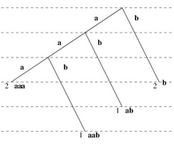## neal young / Golin12Huffman

•We study the generalization of Huffman Coding in which codeword letters have non-uniform costs (as in Morse code, where the dash is twice as long as the dot). Despite previous work by many authors including Richard Karp  and Kurt Mehlhorn , the problem is not known to be NP-hard, nor was it previously known to have a constant-factor approximation algorithm. The paper describes a polynomial-time approximation scheme (PTAS) for the problem. The algorithm computes a $$(1+\epsilon)$$-approximate solution in time $$O(n + f(\epsilon) \log^3 n)$$, where $$n$$ is the input size.
Journal version of .